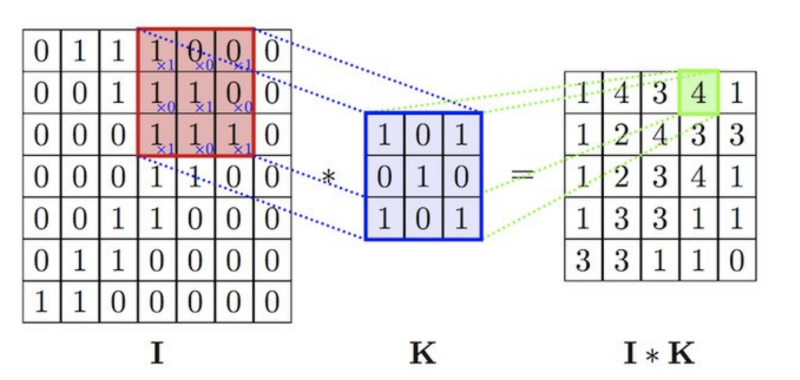# Understanding Convolution Neural Networks -Part I

Source: Deep Learning on Medium# Understanding Convolution Neural Networks -Part I

In this article I will explain the main blocks used in building Convolution Neural Networks and will then proceed to build convolution neural networks from scratch. CNNs have proven to work quite well in image classification, segmentation, object detection etc. CNNs tends to perform quite well as compared to fully connected neural networks with less number of parameters. Let’s define some terms which we would be using in this article.

f = filter size

n_filers = number of filters

s = stride

Why CNNs ?

In Figure 1.1, let’s say we have filter size of 3 and number of filters are 10. The output volume would be of size 30 x 30 x 10. Total number of parameters in this convolution layers are [3 x 3 +1] x 10 = 100. Now let’s say if this was a fully connected neural network, the number of parameters would be [32 x 32 x 3] x [30 x 30 x 10] ≃ 27 Million. That’s a lot of parameters to train and moreover its computationally expensive. Convolution layer has small number of parameters due to parameter sharing and sparsity of connections. Parameter sharing means that imagine a filter that is responsible for detecting vertical edges in the image, during the convolution operation that same filter would slide over the whole image looking for vertical edges. This means that a feature detector such as in this case vertical edge detector that’s useful in one part of the image is probably useful in another part of the image as well so the weights are shared. Sparsity of connections means that In each layer each output value depends only small a small number of inputs as shown in Figure 1.2. This makes CNNs more prone to overfitting and can be trained with smaller training sets.

In Figure 1.2 during the convolution operation, the value 4 in the output image is dependent upon only the 9 values in the red window in the input image. This is known as sparsity of connections.

Convolution Layer

In convolution layer, the convolution filter goes over the image and computes a matrix (element wise product of pixels in image with the convolution window over the image). Convolution operation shrinks down the image and the pixels at the border of the image are used only once in the output as they are not overlapped in the convolution window. This may lead to loss of information from the edges of the image. To avoid this problem padding is used. The hyper parameters of convolution layers are: padding, stride and filter size.

In Figure 1.3, the input image section of size 3 x 3 is convolve with a filter size of 3 x3, doing element wise multiplication of pixel values at each location. Valid convolution means no padding and same convolution means padding such that the output size is same as input size. By convention in the computer vision convolution filter is usually odd. If padding is same, p = f-1/2, where f is the filter size. Strided convolution means that you move the convolution window over the image by s rows and s columns.